Search Results
• #### Please help!!! Consider the three long, straight parallel wires shown in figure. Find the magnitude and...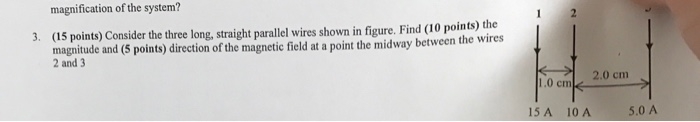Please help!!! Consider the three long, straight parallel wires shown in figure. Find the magnitude and direction of the magnetic field at a point the midway between the wires 2 and 3

• #### Consider the three long, straight parallel wires shown in figure. There is zero magnetic field at...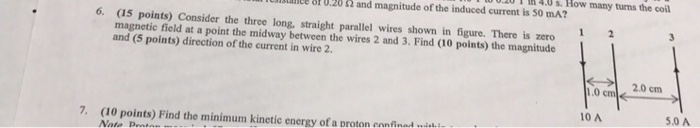Consider the three long, straight parallel wires shown in figure. There is zero magnetic field at a point the midway between the wires 2 and 3. Find the magnitude and direction of the current in wire 2.

• #### Problem 3.24 4 of 8> Part A The wires AB and BC have original lengths of...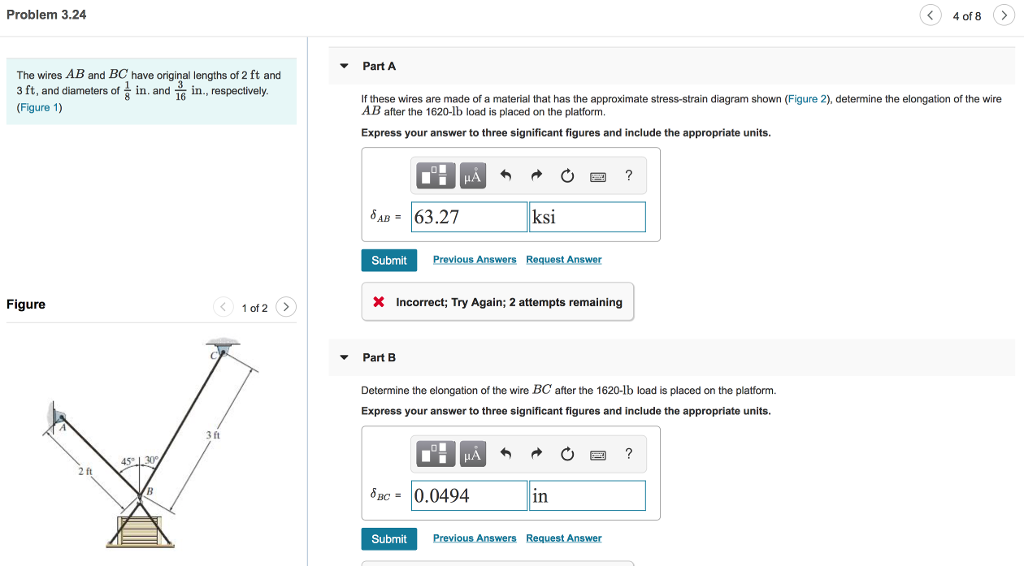Problem 3.24 4 of 8> Part A The wires AB and BC have original lengths of 2 ft and s ft, and diametefn. andin respecivey (Figure 1) 16 If these wires are made of a material that has the approximate stress-strain diagram shown (Figure 2), determine the elongation of the wire AB after the 1620-lb load is placed on the...

• #### Each of the current-carrying wires in the figure (Figure 1) is long and straight, and carries...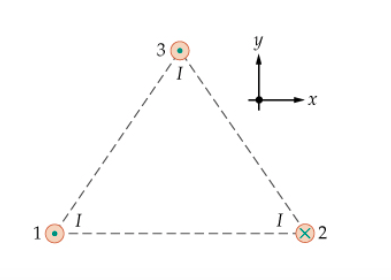Each of the current-carrying wires in the figure (Figure 1) is long and straight, and carries the current II either into or out of the page, as shown. What is the direction of the net magnetic field produced by these three wires at the center of the triangle? 3 у X 1 2

• #### Timing Diagram

How do I draw a timing diagram for figure 2.19a since there are three variables but four wires.

• #### A 6.00-kg ornament is held at rest by two light wires that form 30° angles with...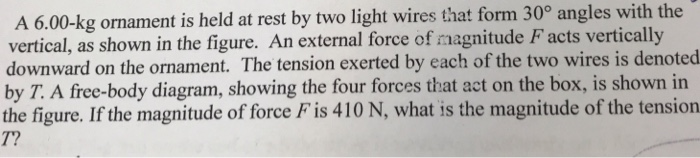A 6.00-kg ornament is held at rest by two light wires that form 30° angles with the vertical, as shown in the figure. An external force of magnitude F acts vertically downward on the ornament. The tension exerted by each of the two wires is denoted by T. A free-body diagram, showing the four forces that act on the box,...

• #### Consider the two cylindrical wires of lengths 41=3.8m and 1=2.2m are merged back to back as...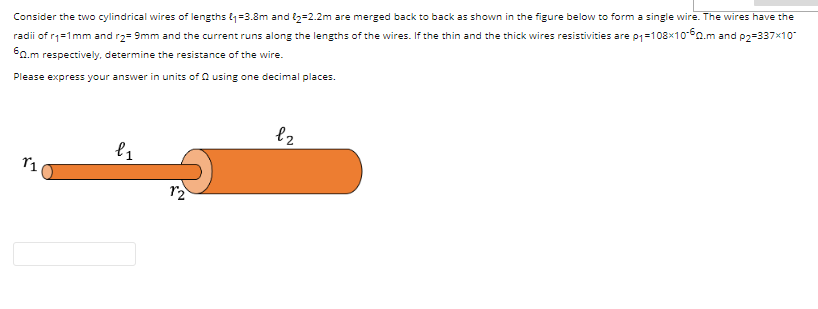Consider the two cylindrical wires of lengths 41=3.8m and 1=2.2m are merged back to back as shown in the figure below to form a single wire. The wires have the radii of r1=1mm and r2=9mm and the current runs along the lengths of the wires. If the thin and the thick wires resistivities are \$1=108x10-60.m and P2=337x10 60.m respectively, determine...

• #### The figure shows the cross section through three long wires with a linear mass distribution of...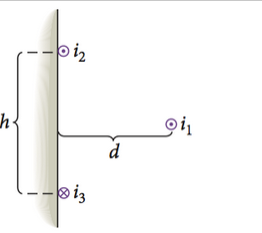The figure shows the cross section through three long wires with a linear mass distribution of 149 g/m. They carry currents i1, i2, and i3 in the directions shown. Wires 2 and 3 are 10.9 cm apart and are attached to a vertical surface, and each carries a current of 687 A. What current, i1, will allow wire 1 to...

• #### Consider a w-N weight suspended by two wires as shown in the accompanying figure. If the...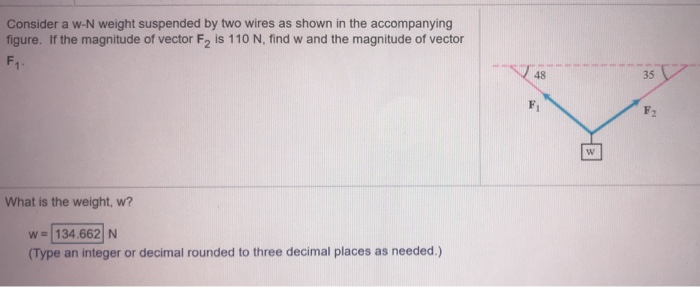Consider a w-N weight suspended by two wires as shown in the accompanying figure. If the magnitude of vector F, is 110 N, find w and the magnitude of vector F1 48 35 F F w What is the weight, w? W = 134.662 N (Type an integer or decimal rounded to three decimal places as needed.)